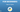# Python Tutorial for Beginners | Tuple in Python### Tuples

Multiple items can be stored in one variable using a tule.

Tuple is one among the 4 data types that Python has built in to store data collections. The other 3 are List and Set. Each type has different properties and uses.

A tuple can be described as a collection that is ordered and unchangeable .

Round brackets are used to write tulles.

Python Tuple consists of a collection separated by commas. A tuple can be compared to a list for its indexing, repetition, and nested objects. However, unlike lists that are mutable, a Tuple is immutable.

#### Creating Tuples in Python

To create a tuple we will use () operators.

mytuple = ("JAVA", "Python", "Kotlin", "NodeJS")
print(mytuple)

### Accessing Values in Tuples in Python

#### Method 1: Using Positive Index

Using square brackets we can get the values from tuples in Python.

mytuple = ("JAVA", "Python", "Kotlin", "NodeJS")

print("Value in mytuple = ", mytuple)
print("Value in mytuple = ", mytuple)
print("Value in mytuple = ", mytuple)
print("Value in mytuple = ", mytuple)

Output

Value in mytuple = JAVA

Value in mytuple = Python

Value in mytuple = Kotlin

Value in mytuple = NodeJS

#### Method 2: Using Negative Index.

In the above methods, we use the positive index to access the value in Python, and here we will use -ve index within [].

mytuple = ("JAVA", "Python", "Kotlin", "NodeJS")

print("Value in mytuple[-4] = ", mytuple[-4])
print("Value in mytuple[-3] = ", mytuple[-3])
print("Value in mytuple[-2] = ", mytuple[-2])
print("Value in mytuple[-1] = ", mytuple[-1])

Output

Value in mytuple[-4] = JAVA

Value in mytuple[-3] = Python

Value in mytuple[-2] = Kotlin

Value in mytuple[-1] = NodeJS

### Concatenation of Tuples in Python

To concatenate the Python tuple we will use plus operators(+).

# Code for concatenating 2 tuples

tuple1 = (0, 1, 2, 3)
tuple2 = ('JAVA', 'Python')

# Concatenating above two
print(tuple1 + tuple2)

Output

(0, 1, 2, 3, ‘JAVA’, ‘Python’)

### Slicing Tuples in Python

# code to test slicing

tuple1 = ("JAVA", "Python", "Kotlin", "NodeJS")
print(tuple1[1:])
print(tuple1[::-1])
print(tuple1[2:4])

Output

(“Python”, “Kotlin”, “NodeJS”) (“NodeJS”, “Kotlin”, “Python”, “JAVA”) (“Kotlin”, “NodeJS”)

### Deleting a Tuple

# code to test slicing

tuple1 = ("JAVA", "Python", "Kotlin", "NodeJS")
del tuple1
print(tuple1)

Output

NameError: name ‘tuple1’ is not defined

### Finding Length of a Tuple

# Code for printing the length of a tuple

tuple1 = ("JAVA", "Python", "Kotlin", "NodeJS")
print(len(tuple1))

Output

4

### Converting list to a Tuple

# Code for printing the length of a tuple

list1 = ["JAVA", "Python", "Kotlin", "NodeJS"]
print(tuple(list1)) #typecasting using tuple keyword

Output

(“JAVA”, “Python”, “Kotlin”, “NodeJS”)